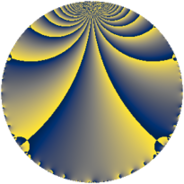Properties

 Label 8280.2.a.yLevel $8280$ Weight $2$ Character orbit 8280.a Self dual yes Analytic conductor $66.116$ Analytic rank $0$ Dimension $2$ CM no Inner twists $1$

Related objects

Newspace parameters

 Level: $$N$$ $$=$$ $$8280 = 2^{3} \cdot 3^{2} \cdot 5 \cdot 23$$ Weight: $$k$$ $$=$$ $$2$$ Character orbit: $$[\chi]$$ $$=$$ 8280.a (trivial)

Newform invariants

 Self dual: yes Analytic conductor: $$66.1161328736$$ Analytic rank: $$0$$ Dimension: $$2$$ Coefficient field: $$\Q(\sqrt{2})$$ Defining polynomial: $$x^{2} - 2$$ Coefficient ring: $$\Z[a_1, \ldots, a_{13}]$$ Coefficient ring index: $$1$$ Twist minimal: no (minimal twist has level 2760) Fricke sign: $$-1$$ Sato-Tate group: $\mathrm{SU}(2)$

$q$-expansion

Coefficients of the $$q$$-expansion are expressed in terms of $$\beta = \sqrt{2}$$. We also show the integral $$q$$-expansion of the trace form.

 $$f(q)$$ $$=$$ $$q - q^{5} - q^{7} +O(q^{10})$$ $$q - q^{5} - q^{7} + ( 2 - 3 \beta ) q^{11} + ( -4 - \beta ) q^{13} + ( 3 + \beta ) q^{17} -5 \beta q^{19} + q^{23} + q^{25} + ( 3 - 3 \beta ) q^{29} + ( -5 - 4 \beta ) q^{31} + q^{35} -3 q^{37} + ( 3 - \beta ) q^{41} + 2 q^{43} + ( -4 - \beta ) q^{47} -6 q^{49} + ( 5 + \beta ) q^{53} + ( -2 + 3 \beta ) q^{55} + ( 5 + 5 \beta ) q^{59} + ( -6 + 5 \beta ) q^{61} + ( 4 + \beta ) q^{65} + ( 5 + 4 \beta ) q^{67} + ( 5 - 5 \beta ) q^{71} + 11 \beta q^{73} + ( -2 + 3 \beta ) q^{77} + 4 \beta q^{79} + ( -9 - 5 \beta ) q^{83} + ( -3 - \beta ) q^{85} + ( 8 + 2 \beta ) q^{89} + ( 4 + \beta ) q^{91} + 5 \beta q^{95} + ( -4 + 2 \beta ) q^{97} +O(q^{100})$$ $$\operatorname{Tr}(f)(q)$$ $$=$$ $$2 q - 2 q^{5} - 2 q^{7} + O(q^{10})$$ $$2 q - 2 q^{5} - 2 q^{7} + 4 q^{11} - 8 q^{13} + 6 q^{17} + 2 q^{23} + 2 q^{25} + 6 q^{29} - 10 q^{31} + 2 q^{35} - 6 q^{37} + 6 q^{41} + 4 q^{43} - 8 q^{47} - 12 q^{49} + 10 q^{53} - 4 q^{55} + 10 q^{59} - 12 q^{61} + 8 q^{65} + 10 q^{67} + 10 q^{71} - 4 q^{77} - 18 q^{83} - 6 q^{85} + 16 q^{89} + 8 q^{91} - 8 q^{97} + O(q^{100})$$

Embeddings

For each embedding $$\iota_m$$ of the coefficient field, the values $$\iota_m(a_n)$$ are shown below.

For more information on an embedded modular form you can click on its label.

Label $$\iota_m(\nu)$$ $$a_{2}$$ $$a_{3}$$ $$a_{4}$$ $$a_{5}$$ $$a_{6}$$ $$a_{7}$$ $$a_{8}$$ $$a_{9}$$ $$a_{10}$$
1.1
 1.41421 −1.41421
0 0 0 −1.00000 0 −1.00000 0 0 0
1.2 0 0 0 −1.00000 0 −1.00000 0 0 0
 $$n$$: e.g. 2-40 or 990-1000 Significant digits: Format: Complex embeddings Normalized embeddings Satake parameters Satake angles

Atkin-Lehner signs

$$p$$ Sign
$$2$$ $$-1$$
$$3$$ $$-1$$
$$5$$ $$1$$
$$23$$ $$-1$$

Inner twists

This newform does not admit any (nontrivial) inner twists.

Twists

By twisting character orbit
Char Parity Ord Mult Type Twist Min Dim
1.a even 1 1 trivial 8280.2.a.y 2
3.b odd 2 1 2760.2.a.q 2
12.b even 2 1 5520.2.a.bk 2

By twisted newform orbit
Twist Min Dim Char Parity Ord Mult Type
2760.2.a.q 2 3.b odd 2 1
5520.2.a.bk 2 12.b even 2 1
8280.2.a.y 2 1.a even 1 1 trivial

Hecke kernels

This newform subspace can be constructed as the intersection of the kernels of the following linear operators acting on $$S_{2}^{\mathrm{new}}(\Gamma_0(8280))$$:

 $$T_{7} + 1$$ $$T_{11}^{2} - 4 T_{11} - 14$$ $$T_{13}^{2} + 8 T_{13} + 14$$ $$T_{17}^{2} - 6 T_{17} + 7$$

Hecke characteristic polynomials

$p$ $F_p(T)$
$2$ $$T^{2}$$
$3$ $$T^{2}$$
$5$ $$( 1 + T )^{2}$$
$7$ $$( 1 + T )^{2}$$
$11$ $$-14 - 4 T + T^{2}$$
$13$ $$14 + 8 T + T^{2}$$
$17$ $$7 - 6 T + T^{2}$$
$19$ $$-50 + T^{2}$$
$23$ $$( -1 + T )^{2}$$
$29$ $$-9 - 6 T + T^{2}$$
$31$ $$-7 + 10 T + T^{2}$$
$37$ $$( 3 + T )^{2}$$
$41$ $$7 - 6 T + T^{2}$$
$43$ $$( -2 + T )^{2}$$
$47$ $$14 + 8 T + T^{2}$$
$53$ $$23 - 10 T + T^{2}$$
$59$ $$-25 - 10 T + T^{2}$$
$61$ $$-14 + 12 T + T^{2}$$
$67$ $$-7 - 10 T + T^{2}$$
$71$ $$-25 - 10 T + T^{2}$$
$73$ $$-242 + T^{2}$$
$79$ $$-32 + T^{2}$$
$83$ $$31 + 18 T + T^{2}$$
$89$ $$56 - 16 T + T^{2}$$
$97$ $$8 + 8 T + T^{2}$$